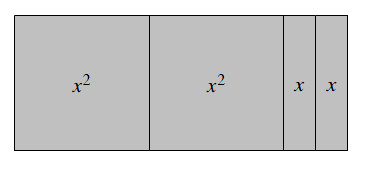### Home > MC2 > Chapter 3 > Lesson 3.1.1 > Problem3-7

3-7.

Sketch the shape made with algebra tiles below on your paper. Then answer parts (a) and (b) below. .

1. Find the area of the shape.

Sum the areas within the shape to find the area of the entire shape.

$x^2 + x^2 + x + x = ?$

$2x^2 + 2x$

2. If the algebra tiles were rearranged into a different shape, how would the area change?

Area is the number of square units needed to fill up a region on a flat surface.

No, the area would not change because the tiles are simply rearranged. This rearrangement of the tiles does not affect the area.Explore the problem with the eTool below.
Click the link at right for the full version of the eTool: MC2 3-7 HW eTool